# MATLAB ODE45 Assignment, Problem 2

The problem: Let's go back to problem 4.4.2 in Strogatz. Numerically solve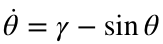for a variety of values of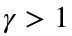. Plot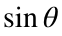as a function of t, and use the time between minima to estimate the period. Try to match the numbers you get to the formula (3) on page 100 of Strogatz, with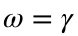and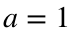.
Here's the problem from Strogatz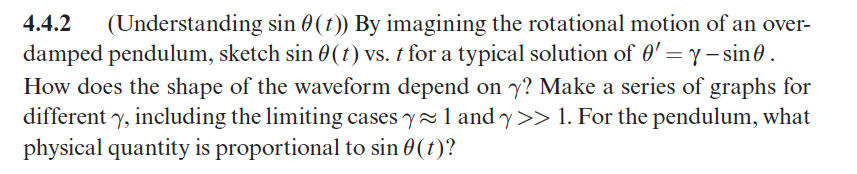Before I start, let's talk about the question of finding the time between minima. I just typed "MATLAB find local minima" into a Google search bar. The first hit is the help page for islocallmin.m. The help page gives me an examplle I can copy to complete the assignment.
Here is my procedure for estimating the period:
1. Simulate the solution for several periods of the motion. I discuss how to choose the final time below. Use a small tolerance to make sure that the time step is small and therefore the times for the minima won't be off by much.
2. Use islocalmin to find an array of minima of. Store the results in an array p. Note that these are indeces of the array theta and not times.
3. Subtract the adjacent elements of t(p) using the diff command.
4. Take the average of these differences using the mean command. This should smooth out any approximation errors.
Notice that the equation we're being asked to simulate is equivalent to equation (1) on p. 98 of the textbook withand. Note that equation (2) tells us that the exact period isand that equation (3) gives us the approximate periodwhen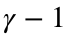is small.
Therefore we will choosed an array of values of γ, which we store in the array gammaVector, and choose the final integration time appropriately large.
Note
gammaVector=1+[1e-3 1e-2 1e-1 1/2 1]; % an array of gamma values that i think will work 1e-6 1e-4 1e-2 1e-1 1/2
nGamma=length(gammaVector);
Texact=2*pi./sqrt(gammaVector.^2-1);
Tapprox=2*pi./sqrt(gammaVector)./sqrt(gammaVector-1);
Tnumerical=zeros(1,nGamma);
options=odeset('RelTol',1e-8,'AbsTol',1e-8);
for k=1:nGamma
tFinal=4*Texact(k);
f=@(t,y)dampedPendulumODE(t,y,gammaVector(k));
[t,theta]=ode45(f,[0 tFinal],0,options);
p=islocalmin(sin(theta));
dt=diff(t(p));
Tnumerical(k)=mean(dt);
figure
plot(t,sin(theta),t(p),sin(theta(p)),'o');
xlabel('$t$');ylabel('$\sin{\theta}$');
title(sprintf('$\\gamma =$%0.3f',gammaVector(k)))
end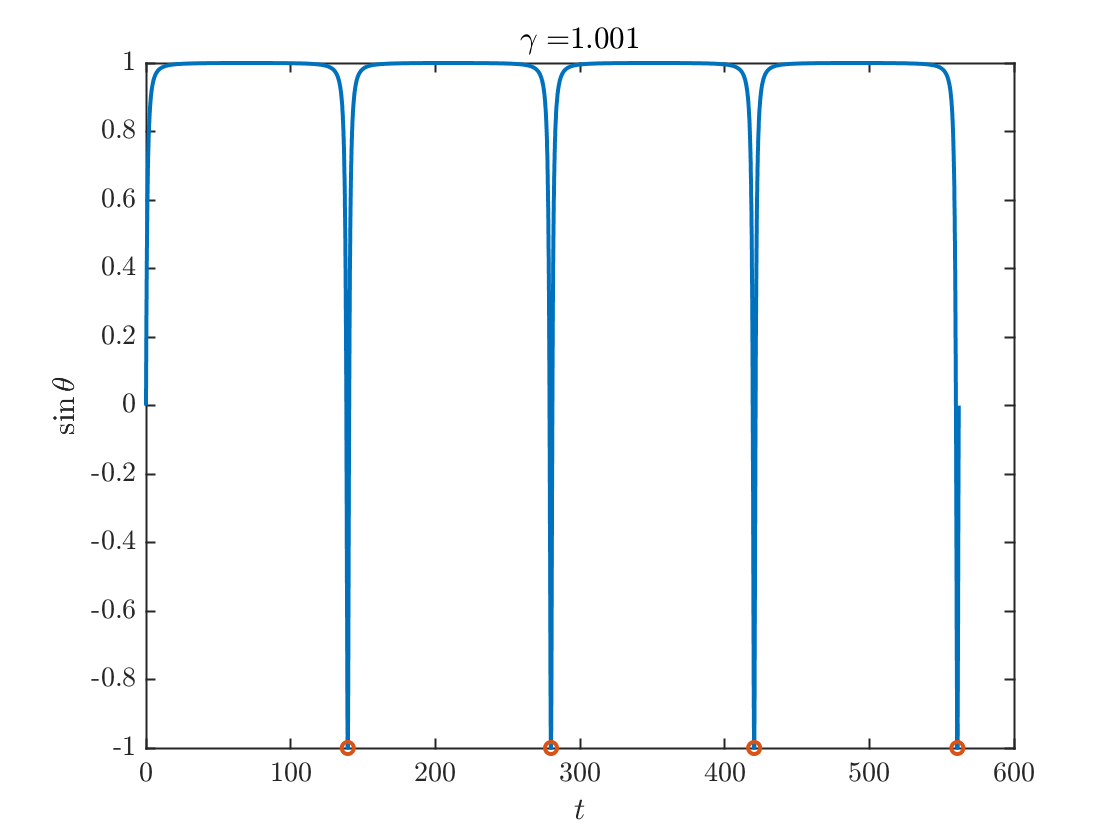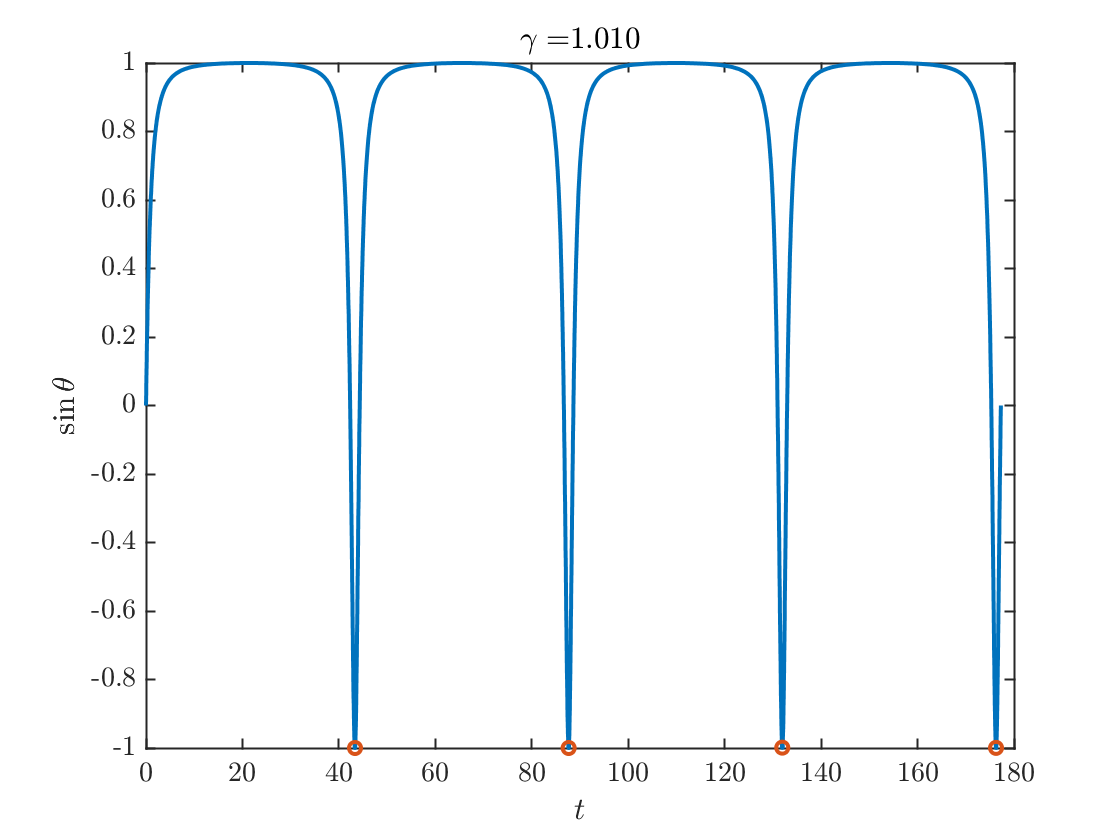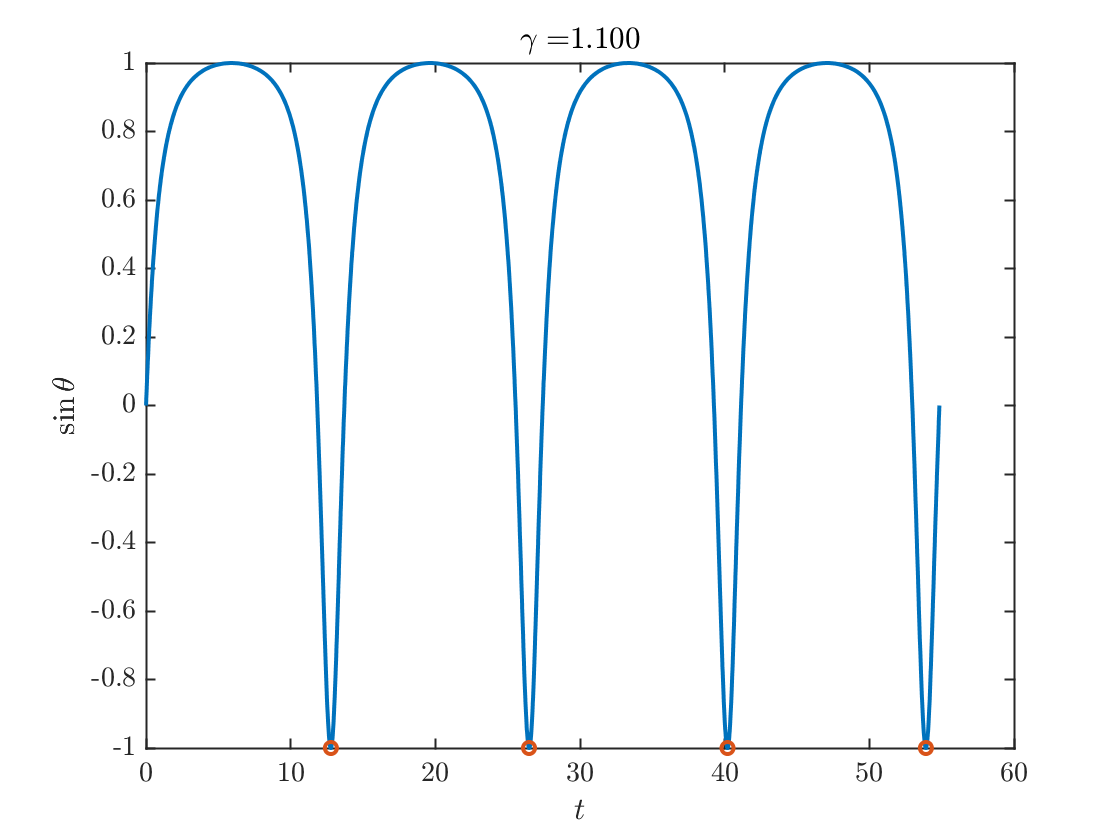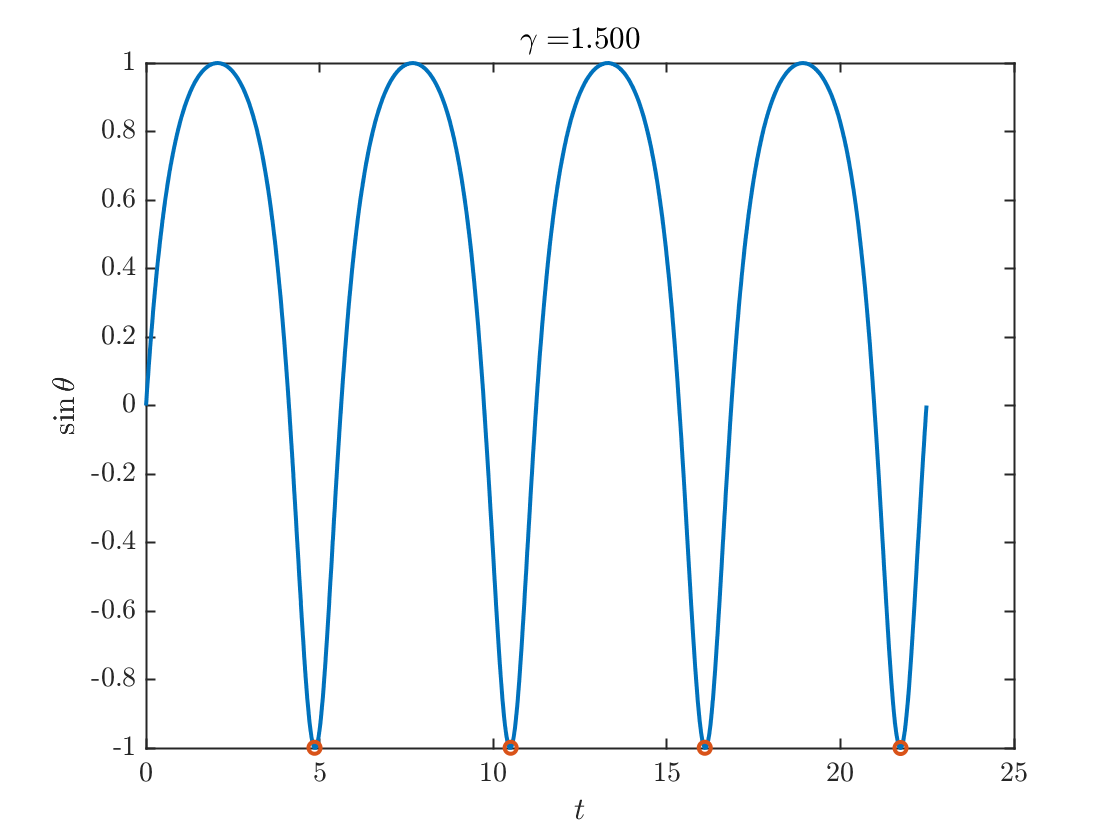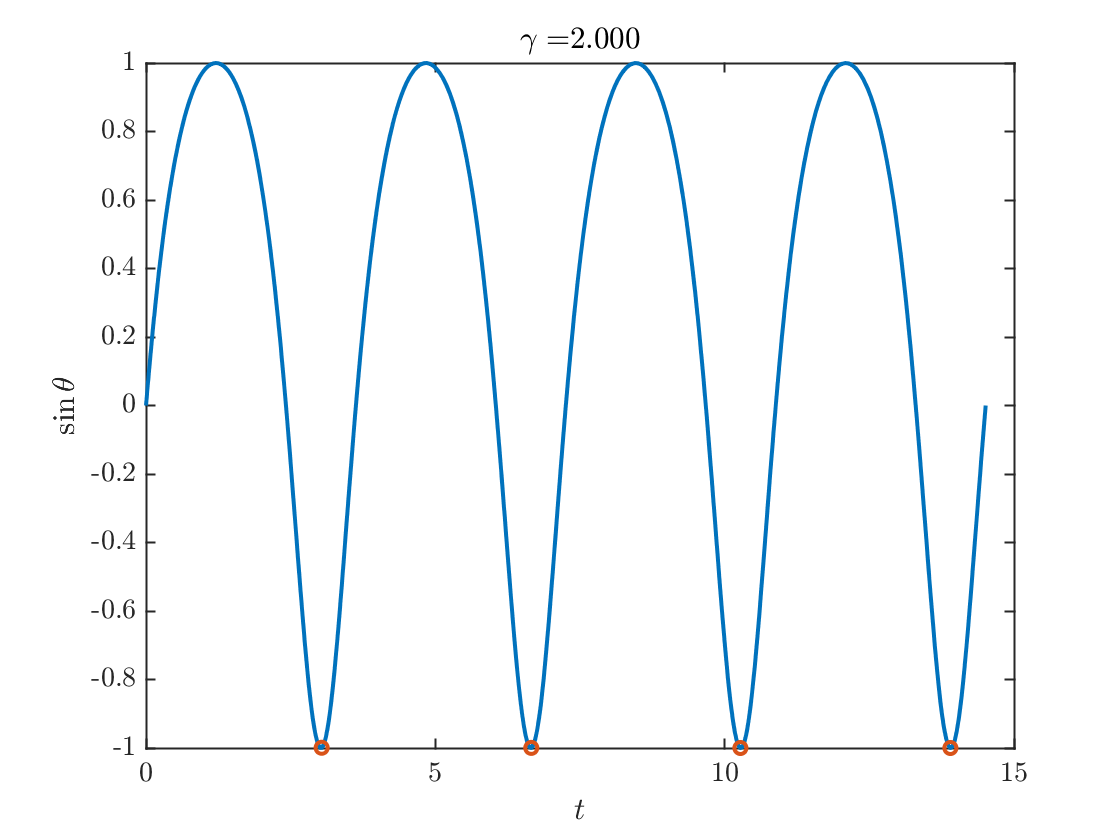Notice the differences in the range of t values on the x-axis.
What we can see from these figures is that the plateaus, i.e., the intervals whereis flat become longer and longer as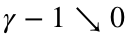.
figure;
loglog(gammaVector-1,Texact,'-o',gammaVector-1,Tapprox,'-x',...
gammaVector-1,Tnumerical,'--o')
legend('Exact Period','Approximate Period','Numerical Period','Location',"best")
xlabel('$\gamma-1$')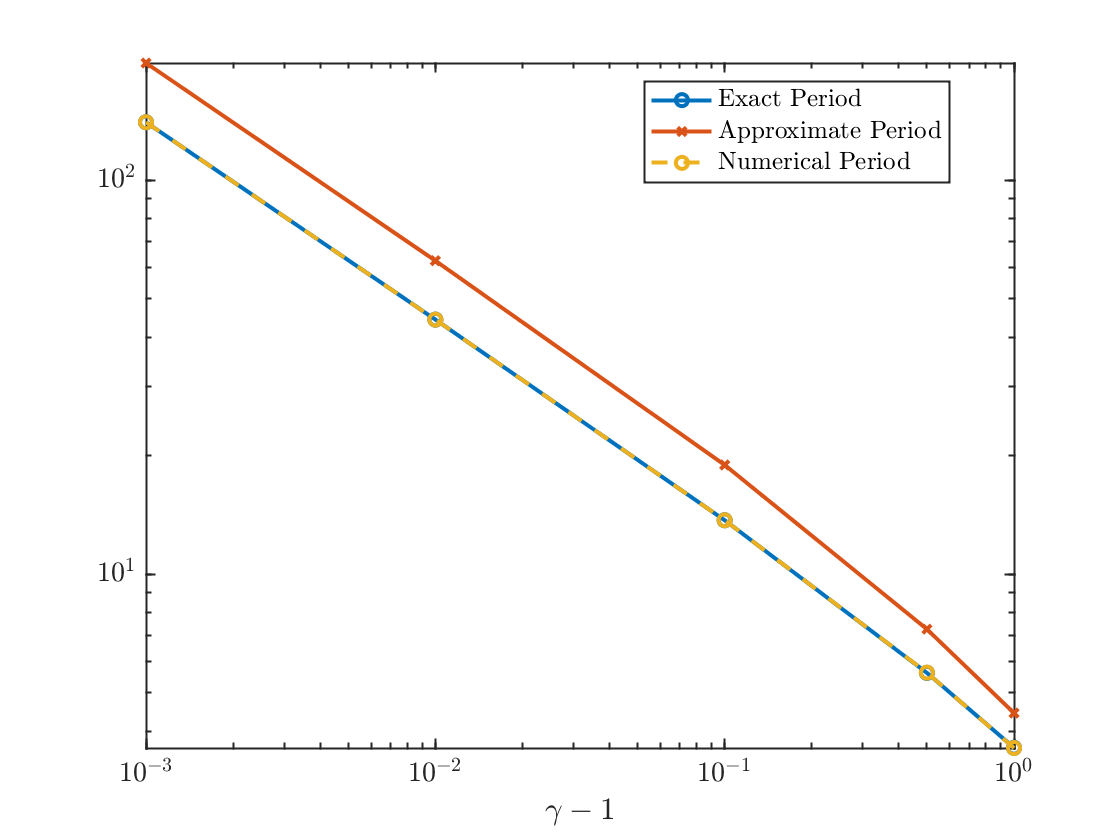We can see that the procedure for finding the minima reproduces the exact formula (2) but that the approximate period from equation (3) appears to be off by a constant whenis small. If the author had kept one more term in his Taylor series when deriving (3), we would see better agreement.
function dydt=dampedPendulumODE(~,y,gamma)
dydt=gamma-sin(y);
end